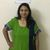# App Development

Announcements
April 22, 2PM EST: Learn about GeoOperations in Qlik Sense SaaS READ MORE
cancel
Showing results for
Did you mean:Creator

## Limited Accumulation in Combo Chart in Qlik Sense

Hello All,

I have a requirement where in I need to use Accumulation in Qlik Sense. Showing the data for completed month ie. June 2019 so my values in MTD from Jan to Dec is coming correct means some numeric values "\$" in Jan to Jun and "0" in Jul to Dec. The similar should happen when doing Accumulation in Column 2 but not possible using either Rowno() or \$(vMonth). Need it in Combo Chart (want Accumulation on Line) but to get correct figures I'm trying it in straight table. Its like values from Jan to Jun: the Line must be raising and from July to Dec: there should be no line. It should stop at June even for Accumulation and should not grow for July and so on. Same when July comes the Line must show for Jan to Jul and rest of the months be "0".

Variables used:

\$(vMonth) = Max(CalendarMonthNumber)

\$(vYearSelection) = Year(Today())

Attached is the reference pic. Formula I'm trying to figure it out:-

1st Column formula - ACT MTD-YTD:

num(Sum({<CalendarMonthNumber={">=1<=\$(vMonth)"},CalendarYear={\$(vYearSelection)}>}[ACT YTD in USD]),'\$#,##0.0')

2nd Column Formula - ACT YTD Acc -1:

Num(RangeSum(Above(Sum({<CalendarMonthNumber={">=1<=\$(vMonth)"},CalendarYear=\$(vYearSelection)},CalendarMonthAndYear=,CalendarMonthName=>}[ACT YTD in USD]),0,RowNo())),'\$#,##0.0')

3rd Column Formula - Acct YTD Acc -2:

num(RangeSum(Above(Sum({<CalendarMonthNumber={">=1<=\$(vMonth)"},CalendarYear={\$(vYearSelection)},CalendarMonthAndYear=,CalendarMonthName=>}[ACT YTD in USD]),0,\$(vMonth))),'\$#,##0.0')

Labels (3)

• ### rangesum

1 Solution

Accepted SolutionsCreator
Author

Thanks a lot for quick reply Sunny but I had nested IF condition and I did something like below:

if(RowNo()>=Num(Month(Today())),0,
if(GetSelectedCount(Currency)>0,
num(RangeSum(Above(
(Sum({<CalendarMonthNumber={">=1<=\$(vMonth)"},CalendarYear={\$(vYearSelection)},CalendarMonthAndYear=,CalendarMonthName=>}[ACT YTD])
),0,RowNo())),'#,##0.0'),
num(RangeSum(Above(
Sum({<CalendarMonthNumber={">=1<=\$(vMonth)"},CalendarYear={\$(vYearSelection)},CalendarMonthAndYear=,CalendarMonthName=>}[ACT YTD in USD])
,0,RowNo())),'\$#,##0.0')))

Coming correct as of now.

Like for July to Dec getting '0' and from Jan to Jun getting '\$ values'
If selecting any currency say 'INR' then again for July to Dec getting '0' and from Jan to Jun getting 'normal values' without \$ that is in local currency.

Do you think what I formulate is the correct solution? Kindly help in validating.

3 RepliesMVP

Try this

``````Num(

RangeSum(Above(Sum({<CalendarMonthNumber, CalendarYear, CalendarMonthAndYear, CalendarMonthName>} [ACT YTD in USD]), 0, RowNo()))

*

Avg({<CalendarMonthNumber={">=1<=\$(vMonth)"}, CalendarYear=\$(vYearSelection)}, CalendarMonthAndYear, CalendarMonthName>} 1)

, '\$#,##0.0')``````Creator
Author

Thanks a lot for quick reply Sunny but I had nested IF condition and I did something like below:

if(RowNo()>=Num(Month(Today())),0,
if(GetSelectedCount(Currency)>0,
num(RangeSum(Above(
(Sum({<CalendarMonthNumber={">=1<=\$(vMonth)"},CalendarYear={\$(vYearSelection)},CalendarMonthAndYear=,CalendarMonthName=>}[ACT YTD])
),0,RowNo())),'#,##0.0'),
num(RangeSum(Above(
Sum({<CalendarMonthNumber={">=1<=\$(vMonth)"},CalendarYear={\$(vYearSelection)},CalendarMonthAndYear=,CalendarMonthName=>}[ACT YTD in USD])
,0,RowNo())),'\$#,##0.0')))

Coming correct as of now.

Like for July to Dec getting '0' and from Jan to Jun getting '\$ values'
If selecting any currency say 'INR' then again for July to Dec getting '0' and from Jan to Jun getting 'normal values' without \$ that is in local currency.

Do you think what I formulate is the correct solution? Kindly help in validating.MVP

If it gives you what you want, then I don't see why it should be not right. On a separate note, did you try what I gave? Did it not work?Tags
Community Browser